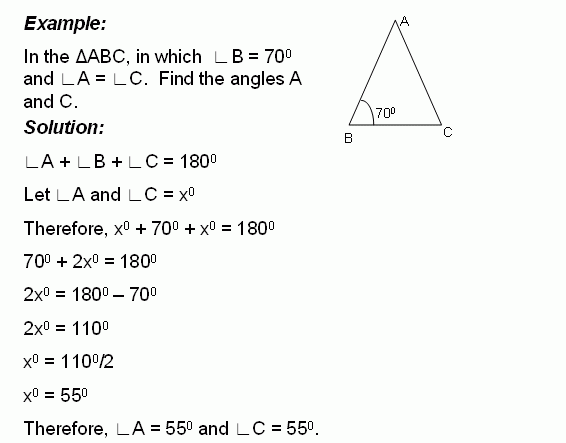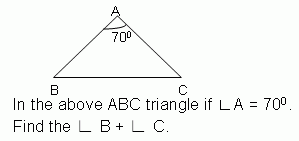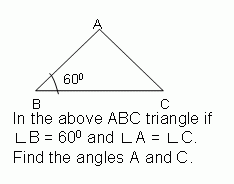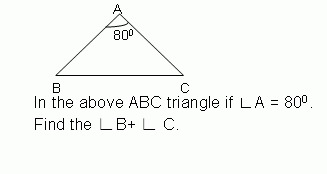Name: ___________________Date:___________________

 Email us to get an instant 20% discount on highly effective K-12 Math & English kwizNET Programs!

### Middle/High School Algebra, Geometry, and Statistics (AGS)6.14 Find the Angles of TriangleDirections: Solve the following problems. Also write at least 10 examples of your own.
 Q 1: The measure of angle x is _____ degrees.Answer: Q 2:105 degrees110 degrees100 degrees Q 3: Find the angles of the triangle and classify based on the angles.26, 64, right angle triangle32, 96, right angle triangle30, 60, right angle triangle Q 4:90 degrees100 degrees95 degrees Q 5:55 degrees60 degrees45 degrees Q 6: Find the angles of the triangle and classify based on the angles.45, 45, 9030, 60, 9020, 60, 100 Q 7:100 degrees160 degrees75 degrees Q 8:60 degrees75 degrees65 degrees Question 9: This question is available to subscribers only! Question 10: This question is available to subscribers only!